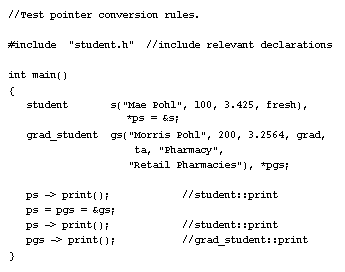Inheritance/Polymorphism  «Prev  Next»
 Lesson 8 A derived class Objective Example of typing conversion and visibility

# Typing Conversion Example in C++

Write input member functions for the student and grad_student classes that read input data for each data member of the respective classes.
The print() member function is implemented in both the student and grad_student classes as follows:

```void student::print() const
{
cout << "\n" << name << " , " << student_id
<< " , " << y << " , " << gpa << endl;
}

{
student::print(); //base class info printed
cout << dept << " , " << s << '\n'
<< thesis << endl;
}
```

For grad_student::print() to invoke the student::print() function, the scope resolved identifier student::print() must be used. Otherwise, there will be an infinite loop. To see which versions of these functions get called, and to demonstrate some of the conversion relationships between base and publicly derived classes, we write a simple test program:

## C++ Test pointer components

The diagram below details the elements of the C++ Test Pointer.This function declares both objects and pointers to them. The conversion rule is that a pointer to a publicly derived class may be converted implicitly to a pointer to its base class. In our example, the pointer variable ps can point at objects of both classes, but the pointer variable pgs can point only at objects of type grad_student. We wish to study how different pointer assignments affect the invocation of a version of print(). This invokes student::print(). It is pointing at the object "s" of type student. This multiple assignment statement has both pointers pointing at an object of type grad_student. The assignment to ps involves an implicit conversion. This again invokes student::print(). The fact that this pointer is pointing at a grad_student object "gs" is not relevant. This invokes grad_student::print(). The variable pgs is of type pointer to grad_student and, when invoked with an object of this type, selects a member function from this class.
Typing - Conversion Visibility